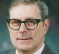## Single vs split HilbertsStarted by 1 year ago1 replylatest reply 1 year ago104 views

I'm trying to get my arms around tradeoffs related to Hilbert filters in IQ SDR radio. It seems that split 45 deg filters can be inherently matched for performance & delay whereas a single 90 deg filter needs to be balanced by a corresponding delay in the opposite channel. Also, the single filter approach seems like it would have more group delay, everything else being equal. Am I correct?

What other factors should be considered?

Joe

[ - ]Joe, I may be beating my own drum here, but I do have some thoughts to share.

First, if an input x(n) is fed into two causal time invariant linear systems, H_1(z) and H_2(z), you won't  get a Hilbert transform of x[n].  You can get the two outputs -- call them x_1(n) and x_2(n) -- to be approximate Hilbert transforms of one another, but x_1(n) and x_2(n) will both have significant group delay relative to x(n).

There may be applications for which that doesn't matter.

Second, we have to distinguish between FIR filters and IIR filters.

You might be looking at a trade-off  between these two cases, with causal recursive filters in both cases.

A) H_1(z) and H_2(z) are both all-pass and their phase difference is approximately 90 degrees. In that case, I would argue that H_1(z) and H_2(z) are matched to one another, in the sense that they are both all-pass and they can't reasonably be expected to also match one another in phase or there wouldn't be any Hilbert transforming going on.

B) H_1(z) and H_2(z) are both all-pass, and they approximate, respectively, a phase of +45 degrees and -45 degrees.  Again, the meaning of matching is not clear. They are both all-pass so they match in amplitude. They certainly don't match in phase.

On the other hand, you might be comparing these other two cases, with causal FIR filters in both cases.

A) H_1(z) and H_2(z) are both FIR filters. H_1(z) is a simple K sample delay. H_2(z) has a perfect 90 degree phase shift  accomplished by making its impulse response odd around a delay of K.    H_2(z)has an additional linear phase z^(-K) to match the delay of H_1(z).

B) H_1(z) and H_2(z) are both FIR filters with identical envelopes. I have never tried that.

I am somewhat prejudiced against the use of FIR Hilbert transformers because they are costly to implement.

Here comes the beating of my own horn. You can design an elliptic phase splitting network, with two outputs H_1(z) and H_2(z),  both all-pass recursive filters with a phase difference approximating 90 degrees, very efficient in  terms of number of multiplications per sample. But you can then change this design to a single non-causal filter with a 90 degree phase shift. Simply interchange the poles and zeroes of H_2(z) with one other, making it H_2^(-1)(z),  and feed H_1(z)'s output into H_2^(-1)(z)'s input. Then the output of H_2(z) is the desired Hilbert transform of the input, except that you will need to delay both the input and the output of H_2^(-1)(z) because H_2^(-1)(z) is not causal.

How can an non-causal filter be implemented? Surprisingly easily. Partition the output of H_1(z) (which is causal recursive etc.) into successive contiguous non-overlapping blocks. Each block has R samples. Each block gets time-reversed, and the time-reversed block is filtered by the original H_2(z)  and after the last of R samples in the block has been used, continue running the filter with a zero input until it has decayed so much that it can be neglected. That decay is exponentially fast.  It's therefore an efficient recursive implementation of a non-recursive filter.  You then have to undo the time-reversal of each of the blocks and add the tail of each block ( the samples past the Rth) to the following block. where they overlap.

This is important. When you design the original pair H_1(z) and H_2(z), choose a design parameter N which is odd. H_1(z) and H_2(z) will then each be functions of  z^2, except that H_1(z) will have an additional factor of z^{-1}.  Being a function of z^2 means that instead of N multiplications per sample, you will only need (N-1)/2 multiplications per sample.   Furthermore you only need to compute half of the samples (saving a second factor of two) because the output plus j times its Hilbert transform is a single sideband and thus has half the bandwidth of the original input x(n). ( I patented this idea long ago, but the patent has long since expired.)